# What Is Xavier Initialization?

As humans, we exercise regularly to stay in shape. In technical terms, we optimize our bodies by training and gaining muscle mass. The same can be said for machine learning and deep learning models. A model like a neural network requires a large amount of training to reach optimal performance levels. That’s why it’s important not to skip specific steps, such as initializing your model with techniques like the Xavier initialization.

This tutorial introduces you to the concept of initialization, shows you why this process is necessary, and explains what the state-of-the-art Xavier initialization is all about.

## What Is Initialization?

Initialization is a crucial part of machine learning, deep learning, and neural networks.

As mentioned, training is essential. Using clumsy or inappropriate methods results in poor performance—in such cases, even the world’s fastest computer won’t be able to help. They say that the devil is in the details, and this saying is particularly true in weight initialization.

Initialization includes setting the initial values of weights for our models, neural networks, or other deep learning architecture. But should these initial weights matter?

## Why Is Initialization Important?

For starters, an improper initialization would lead to an unoptimizable model.

Choosing the right initializer is an essential step in training to maximize performance. For example, if you go with the Xavier initialization, you must ensure this technique is appropriate for your model. In addition, initializing weighted neural networks also shortens the convergence time and minimizes the loss function. The benefits of initialization are worthwhile.

Let’s illustrate the importance of initialization with an example of a model with a single hidden layer: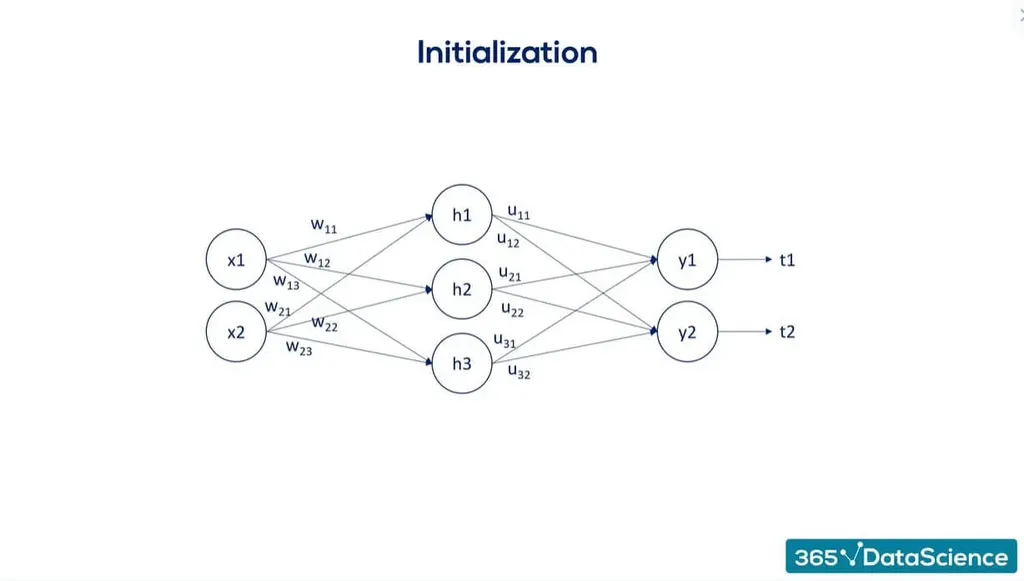Now initialize our weights and biases to equal a constant. (It doesn’t matter which one.)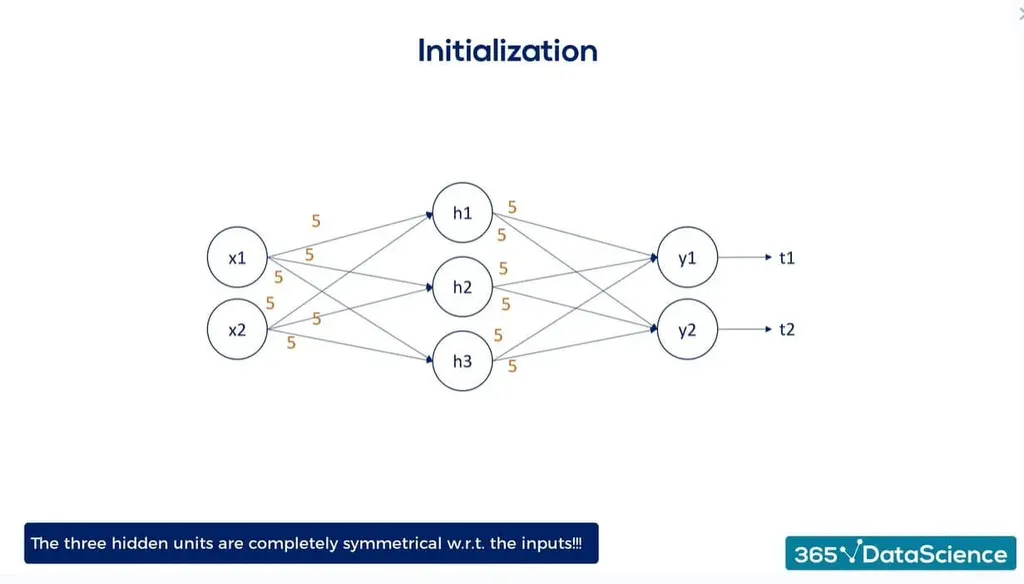As you can see, the three hidden units are entirely symmetrical to the inputs.

Each hidden unit is a function of one weight coming from x1 and one from x2. If all these weights are equal, there’s no reason for the algorithm or neural network to learn that h1, h2, and h3 are different. With forward propagation, there’s no reason for the algorithm to think that even our outputs are different: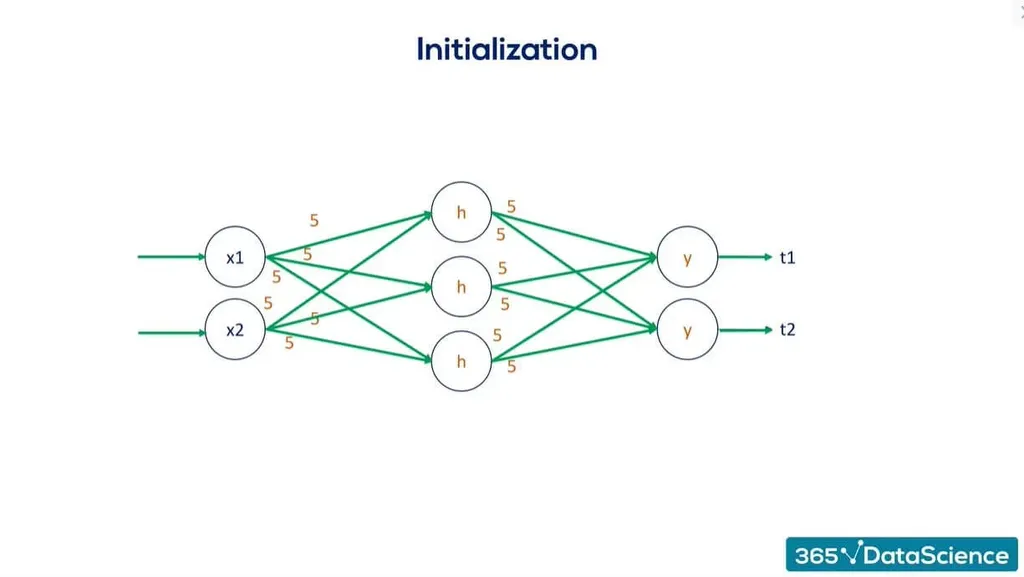Based on this symmetry, when we’re backpropagating, all the weights are bound to be updated without distinguishing between the nodes in the net. Some optimization would still occur, so it won’t be the initial value. Still, the weights would remain useless.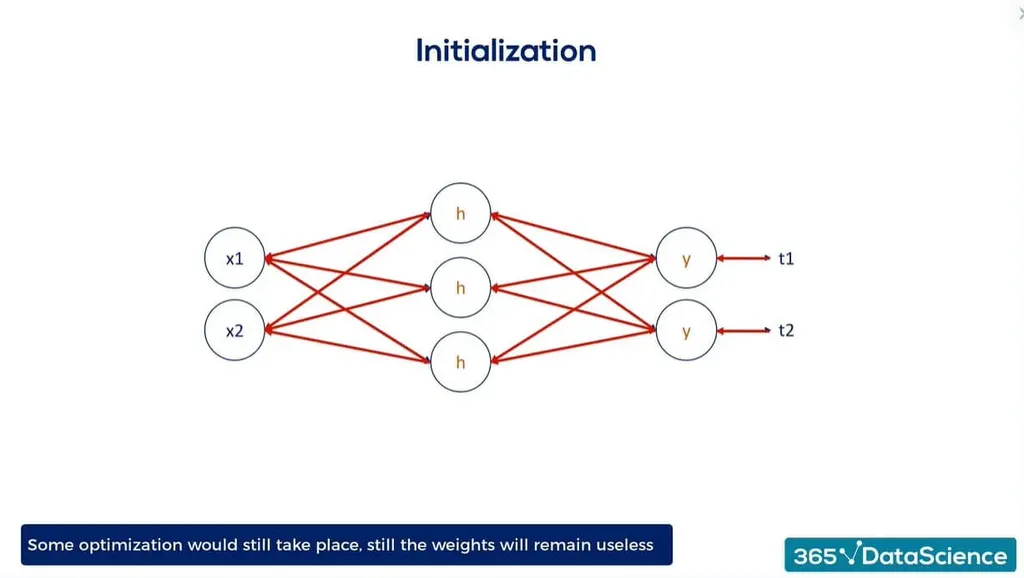We’ll next look at a few types of initialization to determine how to initialize the weights in a neural network.

## Types of Simple Initialization

This article addresses two types of simple initialization: random initialization and normal (naïve) initialization. We’ll also briefly discuss the sigmoid function and its disadvantages in ML models and neural network initialization.

Note: We’ll also discuss the different types of Xavier initialization in the next section, so keep reading to learn more.

Remember, it’s essential to understand what type of initialization method your model needs. Selecting the appropriate technique often requires empirical experimentation and consideration of the specific architecture.

### Random Initialization

A simple approach would be to initialize weights randomly within a small range. We’ll use the NumPy method: random uniform with a range between minus 0.1 and 0.1: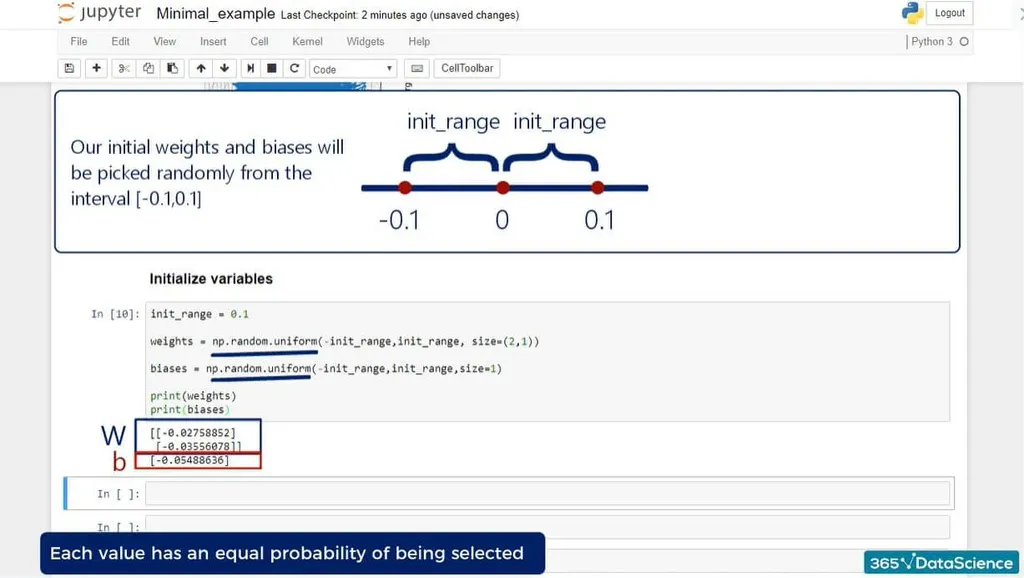Even though the random weight initialization selects the values indiscriminately, they are still chosen uniformly. That means each one has the same probability of being chosen—which may be intuitive, but it’s important to point out.

### Normal (Naïve) Initialization

Another approach is to choose a normal initializer; the idea is the same. But this time, we select the numbers from a zero-mean normal distribution. The chosen variance is arbitrary but should be small. Values closer to 0 are much more likely to be chosen than others.

An example of a normal initialization is to draw from a normal distribution with a mean of 0 and a standard deviation of 0.1: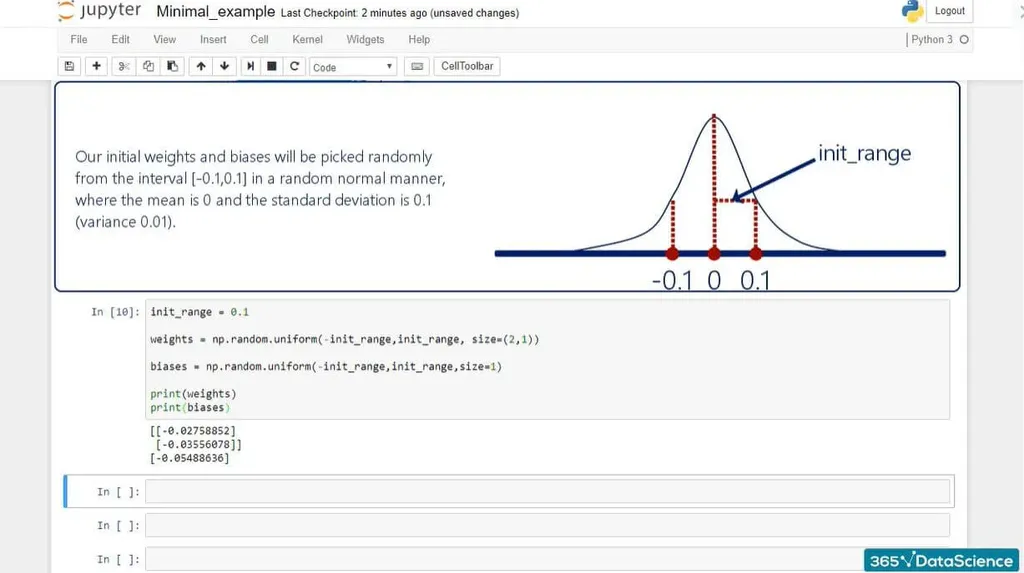Our initial weights and biases will be picked randomly from the interval [-0.1, 0.1] in a usual random manner, where the mean is 0 and the standard deviation is 0.1 (variance 0.01).

Although they were the norm until 2010, both methods could be problematic because they use the sigmoid function. It was only recently that academics came up with a solution.

### What Are the Disadvantages of the Sigmoid Function?

The following example illustrates why sigmoid could be bad for your model or neural network. Weights are used in linear combinations, which we then activate: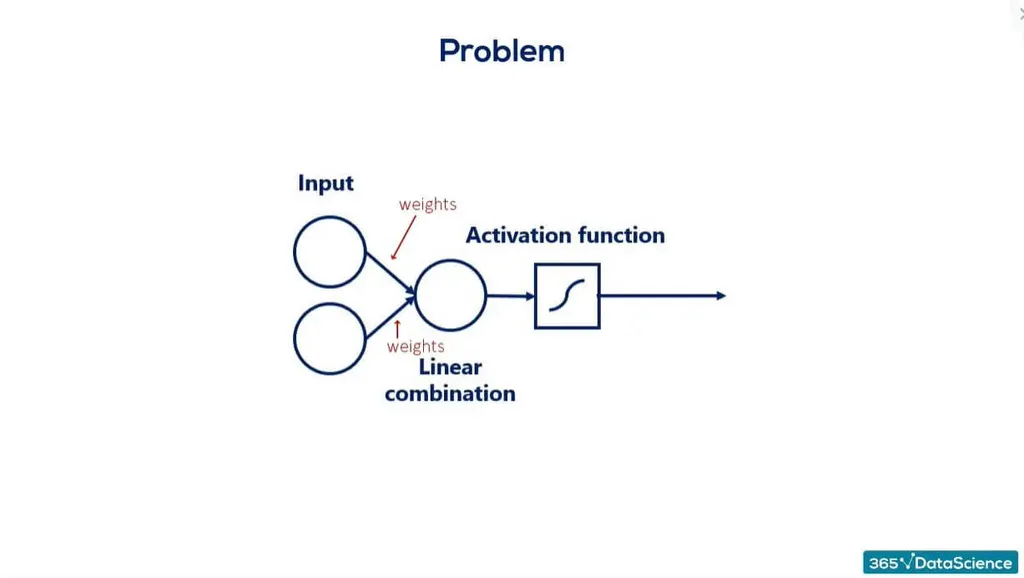In this case, we use the sigmoid activator. This function—like other commonly used non-linearities—is peculiar around its mean and extremes: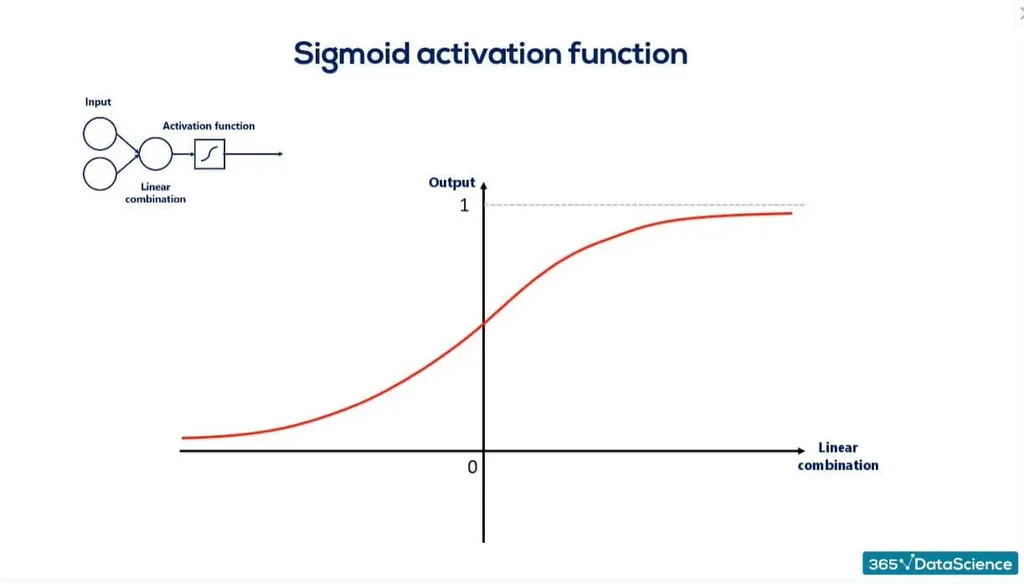Activation functions take the linear combination of the units from the previous layer as inputs. If the weights are too small, this will cause values that fall around this range: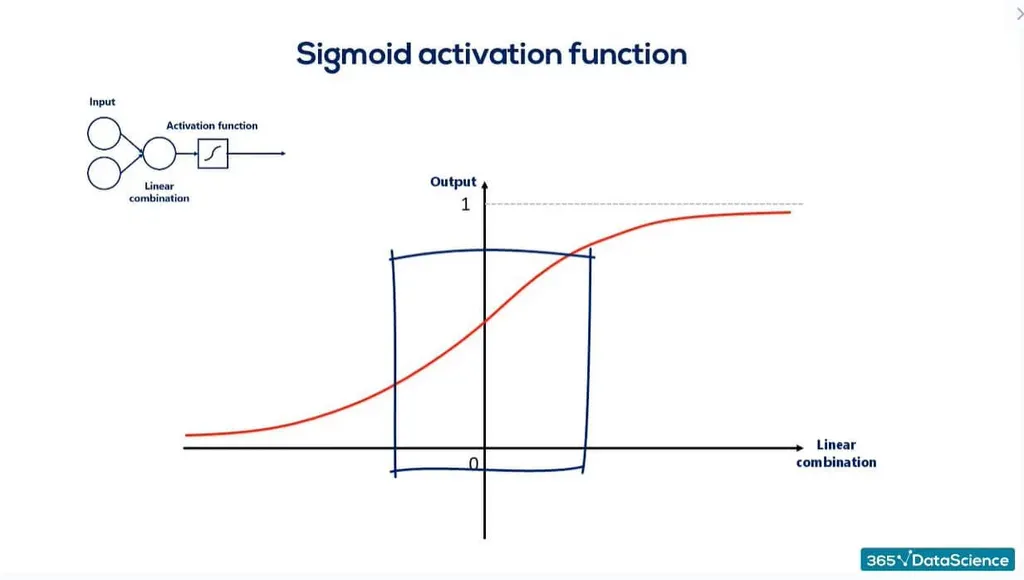As you can see, unfortunately, the sigmoid is almost linear. If all our inputs are in this range—which will occur if we use small weights—our chosen function won’t apply a non-linearity to the linear combination as we want it to. Instead, it’ll involve linearity: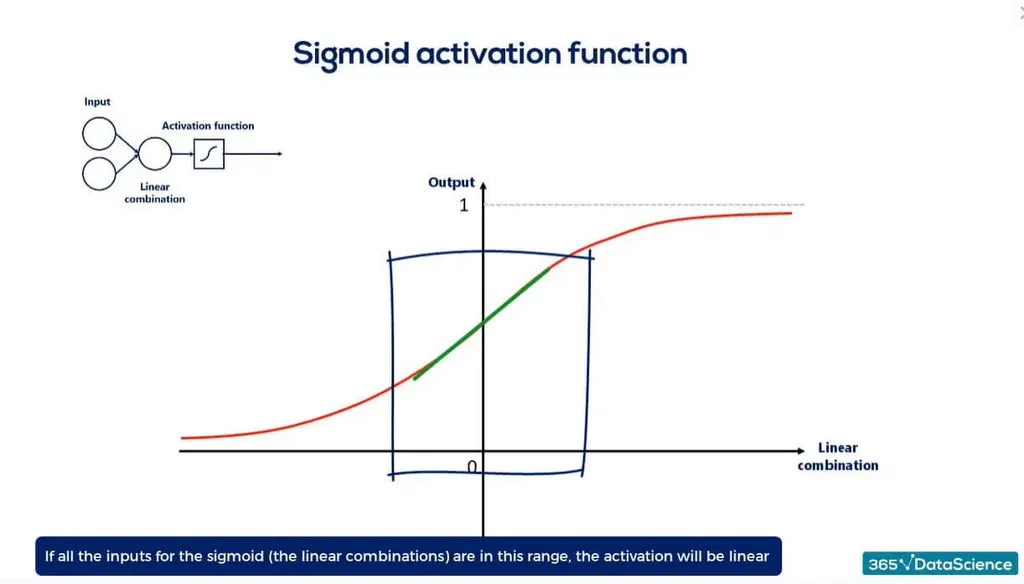Such an outcome is not ideal because non-linearities are essential for deep neural networks.

But if values are too large or too small, the sigmoid would be almost flat—i.e., the output would be only 1s or 0s, respectively: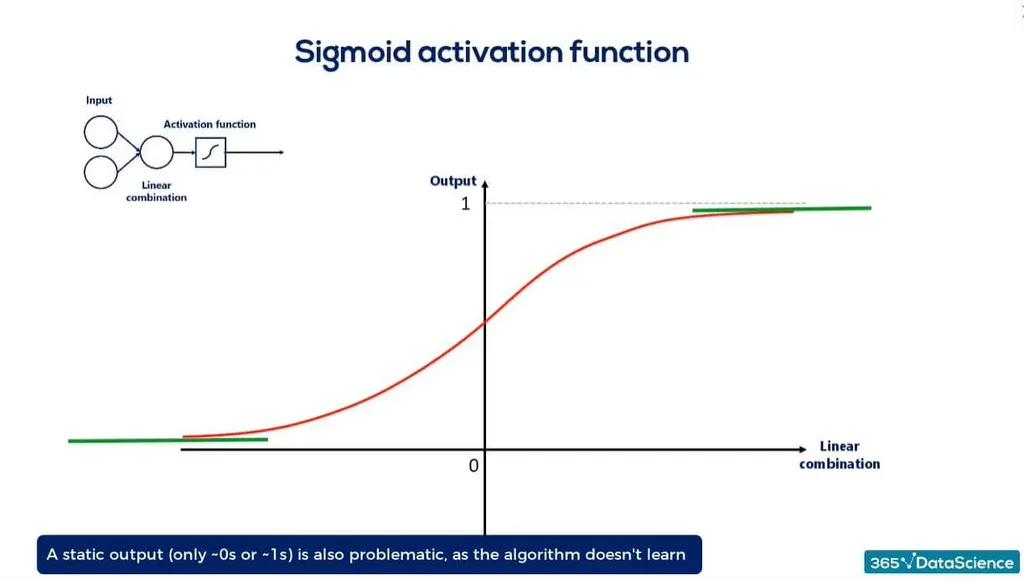A static output of the activations will minimize the gradient while we still haven’t fully trained the algorithm.

So, we want a wide range of inputs for the sigmoid. These inputs depend on the weights, which will have to be initialized in a reasonable range so we can have a nice variance along the linear combinations: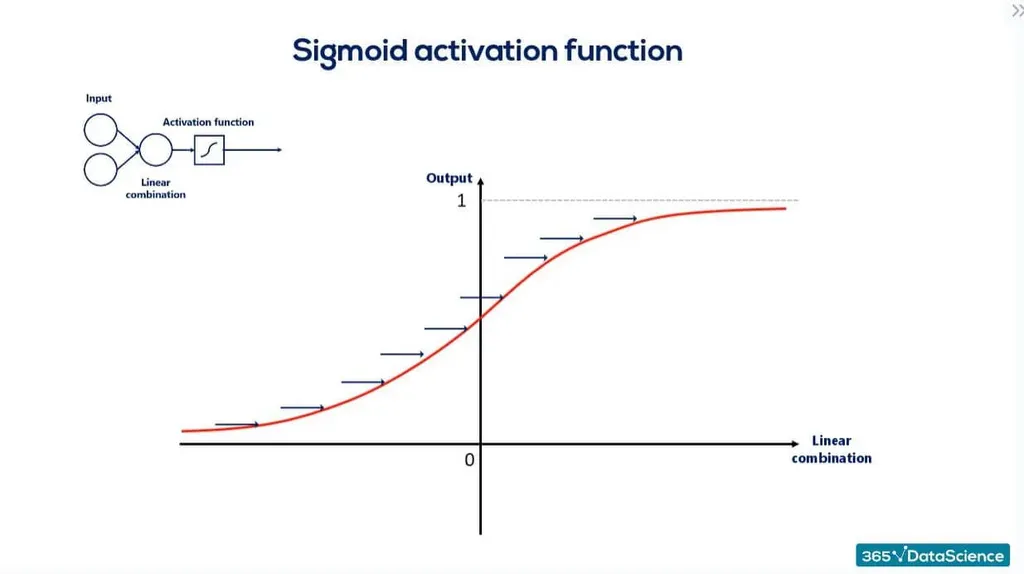We can achieve this by using a more advanced strategy: the Xavier initialization.

## What Is Xavier Initialization?

The Xavier initialization (or Glorot initialization) is a popular technique for initializing weights in a neural network. It’s named after the deep learning researcher Xavier Glorot—the only known academic whose work is named after his first name rather than his last.

Glorot proposed this method in 2010 in his research article Understanding the difficulty of training deep feedforward neural networks. His idea was quickly adopted on a large scale.

The main idea is to set the initial weights of the network in a way that allows the activations and gradients to flow effectively during both forward and backpropagation. It considers the number of input and output units of each layer to determine the scale of the random initialization.

The Xavier initialization is a state-of-the-art technique with which anyone interested in neural networks should be sufficiently acquainted.

Let’s see an example of how this initialization works.

## Example of Xavier Initialization in TensorFlow

We arbitrarily chose the range for the first two cases in our previous example. The Xavier initialization addresses this issue.

The method used for randomization isn’t so important, but rather the number of outputs in the following layer matters. With the passing of each layer, the Xavier initialization maintains the variance in some bounds so that we can take full advantage of the activation functions.

Two formulas are included for this strategy:

Uniform Xavier initialization: draw each weight, w, from a random uniform distribution in in [-x,x] for $x = \sqrt {\frac {6}{inputs\,+\,outputs}}$

Normal Xavier initialization: draw each weight, w, from a normal distribution with mean of 0, and a standard deviation $\sigma = \sqrt {\frac {2}{inputs\,+\,outputs}}$

### Uniform Xavier Initialization

The uniform Xavier initialization—known as the Glorot Uniform in Keras and TensorFlow—states we should draw each weight w from a random uniform distribution in the range from minus x to x, where x is equal to the square root of 6, divided by the number of inputs, plus the number of outputs for the transformation.

### Normal Xavier Initialization

For the normal Xavier initialization, we draw each weight w from a normal distribution with a mean of 0 and a standard deviation equal to 2, divided by the number of inputs plus the number of outputs for the transformation.

The numerator values 2 and 6 vary across sources, but the main idea is the same.

## Why Are Inputs and Outputs in Xavier Initialization Important?

Another detail we should highlight is that the number of inputs and outputs matters when dealing with weighted neural networks and the Glorot initialization method.

Outputs are clear—that’s where the activation function goes. So, the higher the number of outputs, the higher the need to spread weights: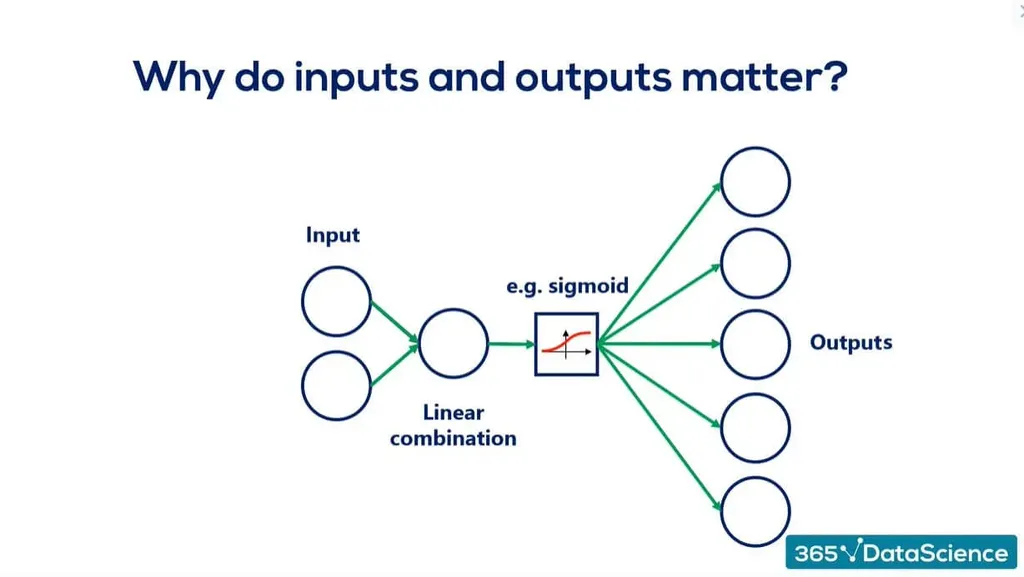What about inputs? Since we achieve optimization through backpropagation, we would have the same problem, but in the opposite direction: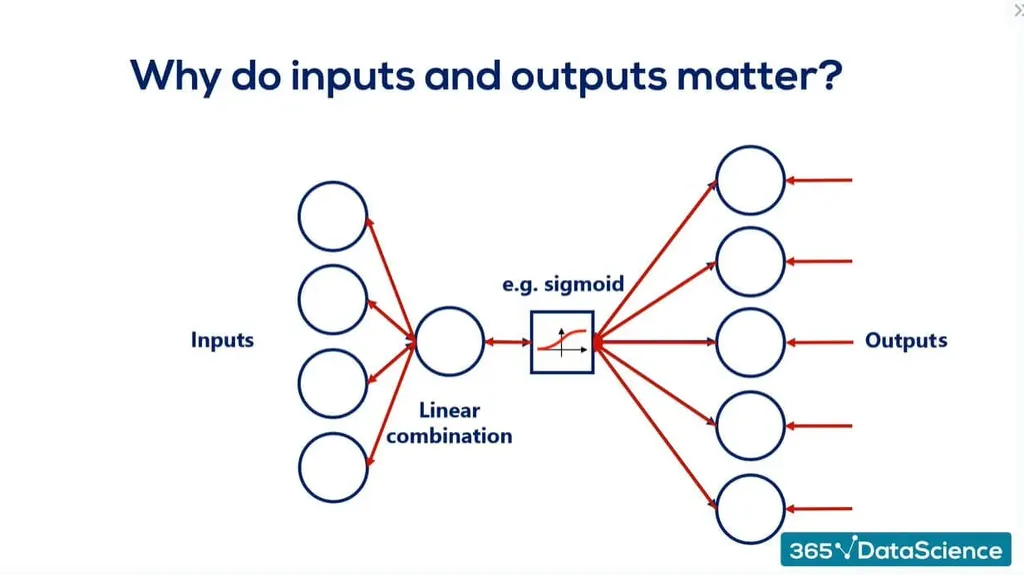Finally, the following is the default initializer in TensorFlow: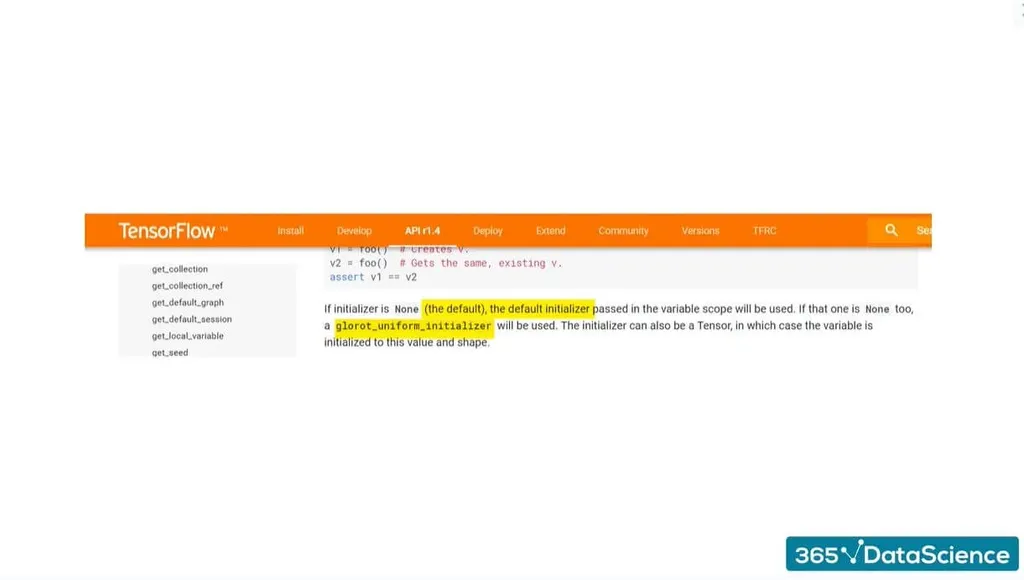So, if you initialize the variables without specifying how to do so, the model will automatically adopt the Xavier initialization.

## Xavier Initialization FAQs

What is the normal Xavier initialization?
Normal Xavier initialization combines the concept of Xavier initialization with a normal (Gaussian) distribution for weight initialization. We draw each weight w from a normal distribution with a mean of 0 and a standard deviation equal to 2, divided by the number of inputs plus the number of outputs for the transformation. The purpose of using a normal distribution in this variation is to introduce more randomness to the weight initialization than a uniform distribution. This can be beneficial sometimes, especially when the neural network is deep or has complex architectures. It's important to note that the choice between Xavier uniform initialization and Xavier normal initialization depends on the specific requirements and characteristics of the neural network.

What is the difference between Xavier and He initialization?
Xavier initialization and He initialization differ in their scaling factors and the activation functions they are designed for. Both initializers address the issue of vanishing or exploding gradients during training. But—depending on the neural network or model—they’re used for different purposes. Xavier initialization considers input and output units and aims to keep the variance of activations and gradients consistent across layers. It works well with the sigmoid activation function, ensuring it’s centered around zero. Meanwhile, the He initialization is designed for the rectified linear unit (ReLU) and its variants. Unlike Xavier, He focuses on input units and provides a more extensive scaling factor to accommodate the characteristics of ReLU activations, which discard negative values.

## Xavier Initialization: Next Steps

As we’ve seen, neural network initialization is a crucial step in training models. Moreover, the Xavier initialization is an innovative method that will save you time and expertly initialize your model’s weights by taking on the brunt of the work. Exploring it as you go along your machine learning and deep learning journey is a good idea. Deep learning is an evolving practice that makes up the backbone of tech innovations and breakthroughs in AI. Staying on top of the trends and following the adopted best practices will ensure you’re on the right track.

Thankfully, you’ve arrived at the best place to start. With high-level training by leading industry experts and a program trusted by over 2M students, 365 Data Science is your gateway into data, machine learning, and AI. Start with a free selection of lessons from our Deep Learning in TensorFlow 2 course and take the first step toward a rewarding tech career.

Learn data science with industry expertsStart your career